﻿ Boffin Access Limited - Open Access Journals - Scholarly Publishing-STEM# ADVANCES IN COMPUTER SCIENCES (ISSN:2517-5718)

### Sacrifice Nana-Kyere1 * , Francis Agyei Boateng1, Glory Kofi Hoggar2, Paddy Jonathan3

1 Department of Mathematics,  Valley View University, Kumasi campus, Ghana
2 Department of Mathematics, Sunyani Technical University, Sunyani, Ghana
3 Department of Mathematics, Osei Kyeretwie Senior High School, Kumasi, Ghana

### CitationCitation COPIED

Kyere SN, Boateng FA, Hoggar GF, Jonathan P. The Stochastic Model. Adv Comput Sci. 2018 Jan; 1(1): 105.

### Introduction

Recent research has revealed a surge in the application of Stochastic Differential Equations (SDEs) in the modeling of infectious diseases. Factors emanating from this surge has been the ability of stochastic differential equations to lay one of the most imperative fundamental differences in the asymptotic dynamics of the deterministic and stochastic epidemic models . These fundamental differences include the convergence of the sample path of stochastic models to the disease-free state and that of the deterministic model to the endemic equilibrium. Stochastic phenomenon occurs naturally in our environment, and when small number of reacting molecules is involved in the modeling system, deterministic models become inappropriate . Stochastic models have properties which include the probability of an outbreak, the quasistationary probability distribution, the final size distribution of an epidemic and the expected duration of an epidemic that makes it unique from others . Stochastic Processes can have moments and covariance function associated with them, which are functions of time, as do random variables .

Stochastic epidemic models have been studied extensively, and the outcomes have been astonishing as they have provided an alternative means of investigating epidemic outbreaks in a small populations coupled with better elucidating of the transmission dynamics of epidemic outbreaks in the early stages of an epidemic outbreak, insinuating the robustness of the deterministic model with respect to stochastic perturbations . Kiouach and Omari , considered an epidemic model of type SIRS and the stability of the interior equilibrium point was discussed. The model was modified to stochastic version and the stability properties of the stochastic model were investigated. Maroufy et al , studied a classical model of a SIRS epidemic in an open population. They deduced an explicit formula by which the lower bound of the density of the infective can be computed. The Model was then extended to stochastic version and Lyapunov functional was employed to investigate the global stability of both the deterministic and the stochastic model. Lahrouz et al , presented an epidemic SIRS model with saturated incidence rate and disease-inflicted mortality. The model was modified into stochastic version and the global stability of the models were studied using the tool of Lyapunov function. Further, the global existence and positivity of the solution was verified as well as the global stability in probability proved under suitable condition by the perturbation of white niose. Murkherjee , considered deterministic and stochastic models for prey-predator system where the prey population is infected by micro parasite. The stochastic stability properties of the models were investigated which suggested the robustness of the deterministic model to stochastic fluctuations. Clancy , formulated a stochastic SIS epidemic model for transmission of infectious disease through a population, considering direct host-host transmission and indirect transmission through free-living infectious stages. Guoting and Tiecheng , investigated a stochastic version of the SIR model. The stability in probability of the steady state of the system was proved under favorable conditions with white noise perturbations. Mukherjee et al , studied the behaviour of a plant herbivore for both discrete and continuous model with stochastic perturbation. The existence and stability of two fixed points were investigated which suggested local asymptotic stability in probability for the stochastic model for certain strengths of white noise. Bifurcation diagrams and time series plots were obtained for the model.

Entrenched in these works are devotees of outstanding achievements and contributions towards scientific community on the mathematical modeling of infectious diseases. In this section, the dynamics of deterministic model of malaria disease and its stochastic variant are presented. The stochastic version of the model is derived by introducing random perturbation to the equations of system (1). Numerical examples are provided to illustrate the explicit differences between the deterministic model and the stochastic system.

The Stochastic Epidemic Model Formulation

The stochastic model of the deterministic system (1) is obtained by introducing stochastic perturbations in the main parameters of the deterministic system. Thus we permit stochastic perturbations of the variables ${S}_{H},{E}_{H},{I}_{H},{R}_{H},{S}_{V},{E}_{V},{I}_{V}$ aroundtheir values at positive equilibrium E [13-15]. Further, we assume that the white noise of the stochastic perturbations of the variable around values of E are proportional to the distances${S}_{H},{E}_{H},{I}_{H},{R}_{H},{S}_{V},{E}_{V},{I}_{V}$ from ${S}_{E}^{*},{E}_{E}^{*},{I}_{E}^{*},{R}_{E}^{*},{S}_{V}^{*},{E}_{V}^{*},{I}_{V}^{*}$ Hence, the stochastic version of model (1) is given by

$\mathrm{d{E}_{H}=\left[{\pi }_{H}+{\rho }_{H}{R}_{H}-{\sigma }_{V}{\beta }_{H}\frac{{I}_{V}}{{N}_{H}}{S}_{H}-{\mu }_{H}{S}_{H}\right]dt+{Q}_{1}\left({S}_{H}-{S}_{H}^{*}\right)d{B}_{1}\phantom{\rule{0ex}{0ex}}}$

$d{E}_{H}=\left[{\sigma }_{V}{\beta }_{H}\frac{{I}_{V}}{{N}_{H}}{S}_{H}-\left({V}_{H}+{\mu }_{H}\right){E}_{H}\right]dt+{Q}_{2}\left({E}_{H}-{E}_{H}^{*}\right)d{B}_{2}$

$d{I}_{H}=\left[{\nu }_{H}{E}_{H}-\left({\gamma }_{H}+{\mu }_{H}+{\delta }_{H}\right){I}_{H}\right]dt+{Q}_{3}\left({I}_{H}-{I}_{H}^{*}\right)d{B}_{3}$

$d{S}_{V}=\left[{\pi }_{V}-{\sigma }_{V}\left(\frac{{\beta }_{V}{I}_{H}+{\beta }_{VH}{R}_{H}}{{N}_{H}}\right){S}_{H}-{\mu }_{V}{S}_{V}\right]dt+{Q}_{5}\left({S}_{V}-{S}_{V}^{*}\right)d{B}_{5}$

$d{E}_{V}=\left[{\sigma }_{V}\left(\frac{{\beta }_{V}{I}_{H}+{\beta }_{VH}{R}_{H}}{{N}_{H}}\right){S}_{V}-\left({\nu }_{V}+{\mu }_{V}\right){S}_{V}\right]dt+{Q}_{6}\left({E}_{V}-{E}_{V}^{*}\right)d{B}_{6}$

$d{I}_{V}=\left[{\nu }_{V}{E}_{V}-{\mu }_{V}{I}_{V}\right]dt+{Q}_{7}\left({I}_{V}-{I}_{V}^{*}\right)d{B}_{7}$

With Qi , for = 1, 2 … … … 7 are real constants and Bi = 1, 2, 3, 4, 5, 6, 7 are independent wiener processes.

### Numerical Method for SDEs-Euler-Maruyama

This section focuses on stochastic modeling application and numerical simulations of trajectories. The work employs the fundamental works which allow the development of effective numerical methods for stochastic differential equations (SDEs), as well as providing the basic information necessary to understand the nature of SDEs and how to stimulate them by using the tool of EulerMaruyama method [16-18]. The method has been proved to have a strong global convergence under the assumption that the stochastic differential equation is locally Lipschitz and that the moment of the exact and numerical solution are bounded for some p > 2.

Consider a scaler, autonomous SDE in integral form

$X\left(t\right)={X}_{0}+{\int }_{0}^{t}f\left(s,x\left(s\right)\right)ds+{\int }_{0}^{t}g\left(s,x\left(s\right)\right)dw\left(S\right),0\le t\le T$      (2)
Where f and g are scaler functions and the initial condition X0 is a random variable.

If x(t) is the solution to (2), then the solution x(t) is a random variable that arises when we take the zero step size limit in the numerical sense. Hence, the differential equation form of (2) can be written as

Now, from equation (3), if g= 0 and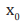is constant, then the problem becomes deterministic and (3) reduces to autonomous ordinary differential equation

(4)

Applying Euler-Maruyama method to (3) over (0, T), we first discretize the interval by letting $\Delta t=\frac{T}{L}$

for some positive integer L and  ${T}_{j}=j\Delta t$. Hence, our numerical approximation to $X\left(T{\right)}_{j}$ will be denoted by ${X}_{j}$. Therefore, the Euler Maruyama (EM) method becomes

(5)

Equation (5) arises from the integral form

(6)

Further, equation (6) reduces to Euler’s method when g = 0 and X0 is constant (see [19-21]).

### Numerical Examples and Discussion

Here, we illustrate with figures the dynamics of the deterministic and stochastic systems, and gives an explicit difference in the models by carrying out numerical simulation for hypothetical set of parameter values. To demonstrate the differences, we simulate the stochastic and the deterministic systems by using the set of parameter values in Table 1. To confirm the deterministic plots, we choose arbitrary white noises  Q1,Q2,Q3,Q4,Q5,Q6 and Q7 of equal strength 0.5, and shows the fluctuations in the trajectories of the stochastic system. We can see that the trajectories of the stochastic plots displayed are the same as the trajectories of the deterministic model during a finite time frame. This suggest that even though fluctuation occurs, they result in the same behavior as the deterministic one. The deterministic approach has limitations that the stochastic handles in a more realistic way. Thus, the deterministic model gives the same results any time the simulation is run with the same parameter and initial values. This might be mathematically correct, however, this is not the case in real epidemic situation, as there may be many parameters which we cannot not model entirely realistically. Modelling them deterministically, we lose some of the complexity of the system. Hence, it is appropriate to assume a stochastic perturbation. Hence, the differences in the dynamics of the models are given by the Figures 1-12.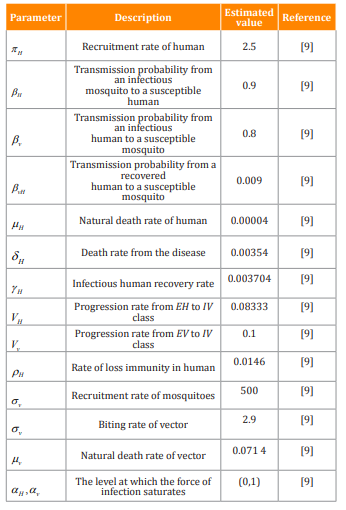Table 1: Description of variables and parameters of the Malaria Model (1)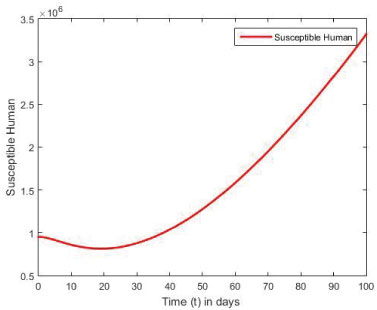Figure 1: The plot represents population of Susceptible Human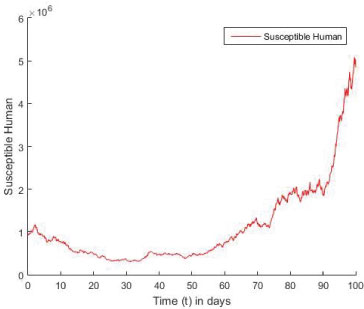Figure 2: Stochastic plot of susceptible Human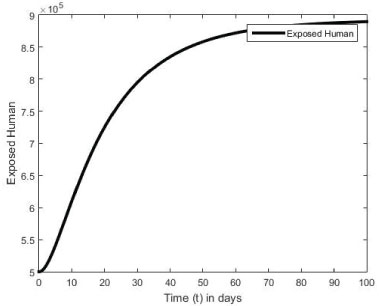Figure 3: The plot represents population of Exposed Human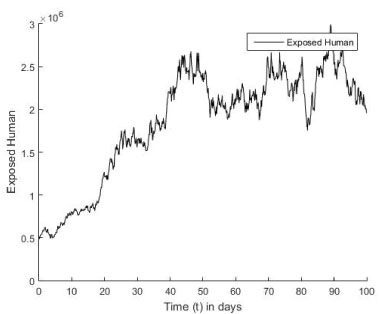Figure 4: Stochastic plot of Exposed Human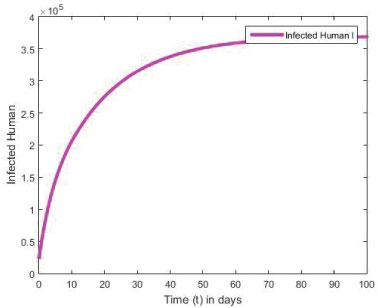Figure 5: The plot represents population of Infected Human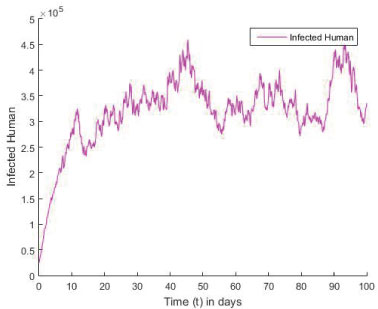Figure 6: Stochastic plot of Infected Human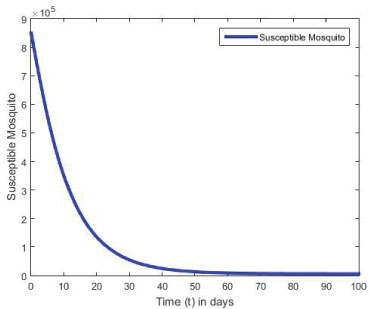Figure 7: The plot represents population of Susceptible Mosquito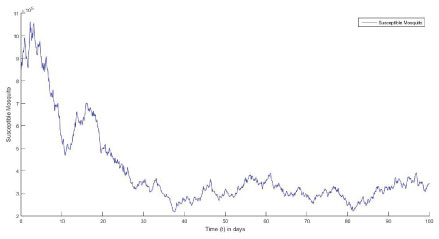Figure 8: Stochastic plot of Susceptible Mosquito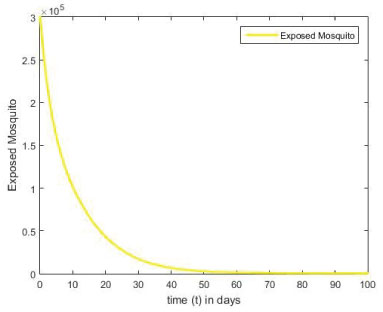Figure 9: The plot represents population of Exposed Mosquito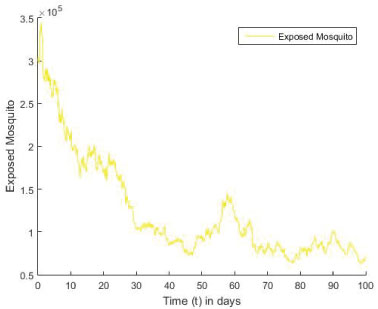Figure 10: Stochastic plot of Exposed Mosquito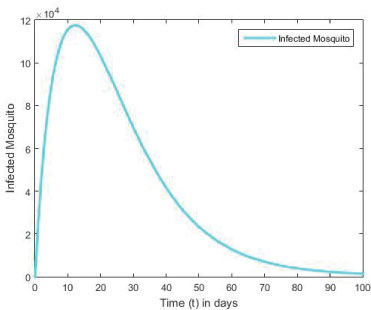Figure 11: The plot represents population of Infected Mosquito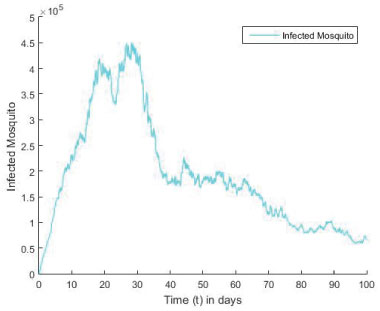Figure 12: Stochastic plot of Infected Mosquito

### Conclusion

In this section, the dynamics of the deterministic malaria model with standard incidence rate and its stochastic version are presented. The stochastic version of the model was constructed by employing stochastic fluctuations in the main parameter of the deterministic model. Our main purpose of the study was to investigate the dynamics of the deterministic and stochastic systems, by giving numerical examples to illustrate the explicit difference in the models. The numerical simulations for the models show that the trajectories of the stochastic plots were the same as the trajectories of the deterministic model. Further, from the stochastic plots, the simulations show an initial random fluctuation of the stochastic trajectories, until they eventually approach asymptotic level. The numerical example shows that the stochastic system is globally asymptotically stable in probability when the densities of white noise are less than certain threshold parameters. However, if these densities of white noise are zero, it means there are no stochastic environmental factors on the population and hence no stochastic perturbation.